# US20220042295A1 - Structural Design Principles For Diagonal Bracings In Truss And Beam Support Systems - Google Patents

Structural Design Principles For Diagonal Bracings In Truss And Beam Support Systems Download PDF

## Info

Publication number
US20220042295A1
US20220042295A1 US17/309,475 US201917309475A US2022042295A1 US 20220042295 A1 US20220042295 A1 US 20220042295A1 US 201917309475 A US201917309475 A US 201917309475A US 2022042295 A1 US2022042295 A1 US 2022042295A1
Authority
US
United States
Prior art keywords
diagonal
beams
structural lattice
lattice
shows
Prior art date
Legal status (The legal status is an assumption and is not a legal conclusion. Google has not performed a legal analysis and makes no representation as to the accuracy of the status listed.)
Pending
Application number
US17/309,475
Inventor
Katia Bertoldi
Matheus C. Fernandes
James C. Weaver
Current Assignee (The listed assignees may be inaccurate. Google has not performed a legal analysis and makes no representation or warranty as to the accuracy of the list.)
Harvard College
Original Assignee
Harvard College
Priority date (The priority date is an assumption and is not a legal conclusion. Google has not performed a legal analysis and makes no representation as to the accuracy of the date listed.)
Filing date
Publication date
Priority to US201862772174P priority Critical
Application filed by Harvard College filed Critical Harvard College
Priority to US17/309,475 priority patent/US20220042295A1/en
Priority to PCT/US2019/063794 priority patent/WO2020113133A1/en
Publication of US20220042295A1 publication Critical patent/US20220042295A1/en
Pending legal-status Critical Current

• 230000002787 reinforcement Effects 0.000 claims abstract description 14
• 239000000463 material Substances 0.000 claims description 26
• 230000000737 periodic Effects 0.000 claims description 25
• 230000003014 reinforcing Effects 0.000 claims description 25
• 230000005540 biological transmission Effects 0.000 claims description 6
• 238000005457 optimization Methods 0.000 description 52
• 238000006073 displacement reaction Methods 0.000 description 27
• 238000007906 compression Methods 0.000 description 21
• 238000000926 separation method Methods 0.000 description 17
• 238000004088 simulation Methods 0.000 description 15
• 238000004458 analytical method Methods 0.000 description 13
• 238000006243 chemical reaction Methods 0.000 description 8
• 238000005259 measurement Methods 0.000 description 5
• 238000005452 bending Methods 0.000 description 3
• 238000002474 experimental method Methods 0.000 description 3
• 239000011159 matrix material Substances 0.000 description 3
• 230000000295 complement Effects 0.000 description 2
• 238000009795 derivation Methods 0.000 description 2
• 230000000694 effects Effects 0.000 description 2
• 230000004048 modification Effects 0.000 description 2
• 238000006011 modification reaction Methods 0.000 description 2
• 239000007787 solid Substances 0.000 description 2
• 238000004450 types of analysis Methods 0.000 description 2
• 238000010146 3D printing Methods 0.000 description 1
• 210000001503 Joints Anatomy 0.000 description 1
• 238000010276 construction Methods 0.000 description 1
• 238000005520 cutting process Methods 0.000 description 1
• 230000001419 dependent Effects 0.000 description 1
• 229920001971 elastomer Polymers 0.000 description 1
• 239000000806 elastomer Substances 0.000 description 1
• 238000005516 engineering process Methods 0.000 description 1
• 238000003698 laser cutting Methods 0.000 description 1
• 230000004301 light adaptation Effects 0.000 description 1
• 239000007788 liquid Substances 0.000 description 1
• 238000004519 manufacturing process Methods 0.000 description 1
• 238000000034 method Methods 0.000 description 1
• 230000002093 peripheral Effects 0.000 description 1
• 238000007639 printing Methods 0.000 description 1
• 238000009877 rendering Methods 0.000 description 1
• 238000001228 spectrum Methods 0.000 description 1
• 238000006467 substitution reaction Methods 0.000 description 1
• 230000001131 transforming Effects 0.000 description 1

## Images

•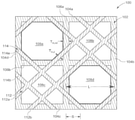•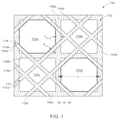••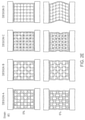•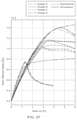•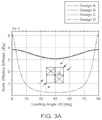•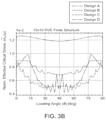•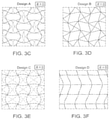•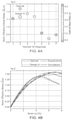••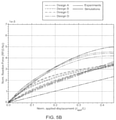•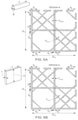•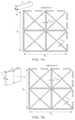•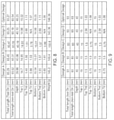•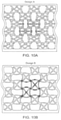•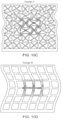•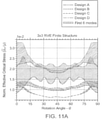•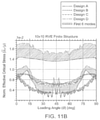•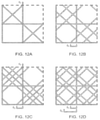••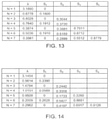•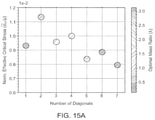•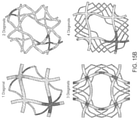•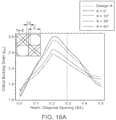•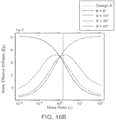•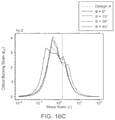•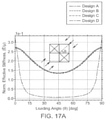•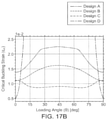•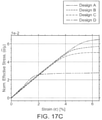•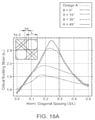•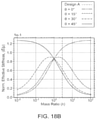•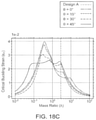•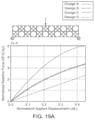••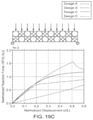•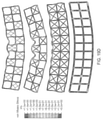•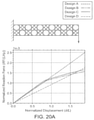•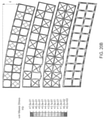••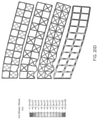•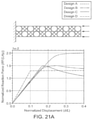••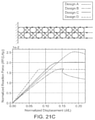•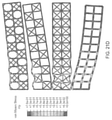•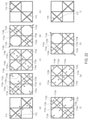•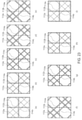••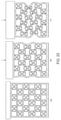•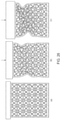•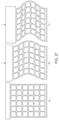## Classifications

• EFIXED CONSTRUCTIONS
• E04BUILDING
• E04BGENERAL BUILDING CONSTRUCTIONS; WALLS, e.g. PARTITIONS; ROOFS; FLOORS; CEILINGS; INSULATION OR OTHER PROTECTION OF BUILDINGS
• E04B1/00Constructions in general; Structures which are not restricted either to walls, e.g. partitions, or floors or ceilings or roofs
• E04B1/18Structures comprising elongated load-supporting parts, e.g. columns, girders, skeletons
• E04B1/19Three-dimensional framework structures
• EFIXED CONSTRUCTIONS
• E04BUILDING
• E04CSTRUCTURAL ELEMENTS; BUILDING MATERIALS
• E04C3/00Structural elongated elements designed for load-supporting
• E04C3/02Joists; Girders, trusses, or trusslike structures, e.g. prefabricated; Lintels; Transoms; Braces
• E04C3/04Joists; Girders, trusses, or trusslike structures, e.g. prefabricated; Lintels; Transoms; Braces of metal
• E04C3/08Joists; Girders, trusses, or trusslike structures, e.g. prefabricated; Lintels; Transoms; Braces of metal with apertured web, e.g. with a web consisting of bar-like components; Honeycomb girders
• BPERFORMING OPERATIONS; TRANSPORTING
• B32LAYERED PRODUCTS
• B32BLAYERED PRODUCTS, i.e. PRODUCTS BUILT-UP OF STRATA OF FLAT OR NON-FLAT, e.g. CELLULAR OR HONEYCOMB, FORM
• B32B3/00Layered products comprising a layer with external or internal discontinuities or unevennesses, or a layer of non-planar form; Layered products having particular features of form
• B32B3/10Layered products comprising a layer with external or internal discontinuities or unevennesses, or a layer of non-planar form; Layered products having particular features of form characterised by a discontinuous layer, i.e. formed of separate pieces of material
• B32B3/12Layered products comprising a layer with external or internal discontinuities or unevennesses, or a layer of non-planar form; Layered products having particular features of form characterised by a discontinuous layer, i.e. formed of separate pieces of material characterised by a layer of regularly- arranged cells, e.g. a honeycomb structure
• EFIXED CONSTRUCTIONS
• E04BUILDING
• E04CSTRUCTURAL ELEMENTS; BUILDING MATERIALS
• E04C3/00Structural elongated elements designed for load-supporting
• E04C3/02Joists; Girders, trusses, or trusslike structures, e.g. prefabricated; Lintels; Transoms; Braces
• E04C3/04Joists; Girders, trusses, or trusslike structures, e.g. prefabricated; Lintels; Transoms; Braces of metal
• E04C3/08Joists; Girders, trusses, or trusslike structures, e.g. prefabricated; Lintels; Transoms; Braces of metal with apertured web, e.g. with a web consisting of bar-like components; Honeycomb girders
• E04C3/083Honeycomb girders; Girders with apertured solid web
• EFIXED CONSTRUCTIONS
• E04BUILDING
• E04CSTRUCTURAL ELEMENTS; BUILDING MATERIALS
• E04C5/00Reinforcing elements, e.g. for concrete; Auxiliary elements therefor
• E04C5/01Reinforcing elements of metal, e.g. with non-structural coatings
• E04C5/06Reinforcing elements of metal, e.g. with non-structural coatings of high bending resistance, i.e. of essentially three-dimensional extent, e.g. lattice girders
• EFIXED CONSTRUCTIONS
• E04BUILDING
• E04CSTRUCTURAL ELEMENTS; BUILDING MATERIALS
• E04C3/00Structural elongated elements designed for load-supporting
• E04C3/02Joists; Girders, trusses, or trusslike structures, e.g. prefabricated; Lintels; Transoms; Braces
• E04C2003/026Braces

## Abstract

A structural lattice includes a rectangular base defined by four periphery beams, and two non-diagonal beams that divide the rectangular base in four quadrants. The structural lattice further includes a diagonal reinforcement strut system overlaid on the rectangular base and having at least two intersecting sets of diagonal beams forming an open-and-closed cell architecture.

## Description

CROSS-REFERENCE TO RELATED APPLICATIONS
• This application claims priority to and benefit of U.S. Provisional Patent Application Ser. No. 62/772,174, filed on Nov. 28, 2018, which is hereby incorporated by reference herein in its entirety.
• STATEMENT REGARDING FEDERALLY SPONSORED RESEARCH
• Some aspects of the present disclosure were made with government support, under NSF Grant No. DGE-1144152 and NSF Grant No. DRMEF-1533985 awarded by the National Science Foundation (NSF), and the government shares rights to such aspects of the present disclosure.
• FIELD OF THE INVENTION
• The present invention relates generally to a structural element, and, more particularly, to a diagonal bracing in beam and truss support systems.
• BACKGROUND OF THE INVENTION
• Structural bracings are typically used in beam and truss support systems commonly employed in buildings, bridges, aerospace applications, automotive applications, and power-transmission application. Additionally, structural bracings are further used in numerous other macro-scale and micro-scale examples.”
• For example, dating back to the 1800s, architects Ithiel Town and Colonel Long introduced complementary and simple designs for diagonal bracings in square grid lattices. Although simple, these early designs have historically proven to be effective in supporting beam structures. Based on that early success, there has been very limited research and little deviation from these old designs in modern engineering structures. As a great disadvantage, in present days the load-carrying capacity of these old designs has reached its limits. Thus, for example, weight presently limits strength and/or height of structures. In another example, weight limits lengths of structures without support, in applications such as bridges.
• Thus, there is a need for providing a structural element that increases strength without increasing weight, and that prevents or reduces the above and other problems.
• SUMMARY OF THE INVENTION
• According to one embodiment of the present disclosure, a structural lattice includes a rectangular base defined by four periphery beams, and two non-diagonal beams that divide the rectangular base in four quadrants. The structural lattice further includes a diagonal reinforcement strut system overlaid on the rectangular base and having at least two intersecting sets of diagonal beams forming an open-and-closed cell architecture.
• According to an implementation of this embodiment, one of the two intersecting sets of diagonal beams is a first set of diagonal beams, which includes first beam that is parallel to a second beam. According to a configuration of this implementation, the first beam and the second beam are symmetrically positioned over one of the four quadrants. According to another configuration of this implementation, another one of the two intersecting sets of diagonal beams is a second set of diagonal beams, the second set of diagonal beams including a respective first beam that is parallel to a respective second beam. According to one example of this configuration, the first set of diagonal beams intersects the second set of diagonal beams at a perpendicular angle. According to another example of this configuration, the respective first beam and the respective second beam are symmetrically positioned over one of the four quadrants. According to an optional aspect of this example, the first beam and the second beam of the first set of diagonal beams are symmetrically positioned over a same one of the four quadrants as the respective first beam and the respective second beam of the second set of diagonal beams.
• According to another implementation of this embodiment, at least one of the four quadrants is an open cell having an equilateral octagon shape. The equilateral octagon shape is defined by two of the four periphery beams, the two non-diagonal beams, and four beams of the at least two intersecting sets of diagonal beams. According to yet another implementation of this embodiment, the rectangular base and the diagonal reinforcement strut system form at least a structural portion of a building, a bridge, an aerospace structure, an automotive structure, or a power transmission structure. According to yet another implementation of this embodiment, the diagonal reinforcement strut system is welded to the rectangular base.
• According to another embodiment of the present disclosure, a periodic structural lattice has a plurality of non-diagonal reinforcing struts forms a base structure of the periodic structural lattice. The base structure is defined by a base periphery, and the plurality of non-diagonal reinforcing struts have a first volume of material. The periodic structural lattice further has a plurality of diagonal reinforcing struts coupled to the base structure and having a predetermined cross-sectional geometry that forms open and closed cells with the plurality of non-diagonal reinforcing struts. The plurality of diagonal reinforcing struts have positive and negative slopes relative to the plurality of non-diagonal reinforcing struts. The plurality of diagonal reinforcing struts is spaced apart at predetermined intervals within the base periphery and has a second volume material. The first volume of material and the second volume of material are less than a total volume of the periodic structural lattice that includes the open and closed cells.
• According to one implementation of this embodiment, the plurality of non-diagonal reinforcing struts have a round cross-section. According to another implementation of this embodiment, the plurality of non-diagonal reinforcing struts have a square cross-section.
• According to yet another implementation of this embodiment, the base periphery has four periphery beams forming a rectangular shape. According to a configuration of this implementation, the four periphery beams have a round cross-section. According to another configuration of this implementation, the four periphery beams have a square cross-section.
• According to yet another implementation of this embodiment, the plurality of diagonal reinforcing struts includes a first pair of parallel beams and a second pair of parallel beams. The first pair of parallel beams intersects the second pair of parallel beams at a predetermined angle. According to a configuration of this implementation, the predetermined angle is 90°.
• According to yet another implementation of this embodiment, the positive and negative slopes are formed by perpendicularly intersecting pairs of the plurality of non-diagonal reinforcing struts. According to yet another implementation of this embodiment, the base structure and the plurality of diagonal reinforcing struts form a repeating sub-unit of at least a structural portion of a building, a bridge, a crane, an aerospace structure, an automotive structure, or a power transmission structure.
• Additional aspects of the invention will be apparent to those of ordinary skill in the art in view of the detailed description of various embodiments, which is made with reference to the drawings, a brief description of which is provided below.
• BRIEF DESCRIPTION OF THE DRAWINGS
• FIG. 1 shows a planar idealization of a Design A structure.
• FIG. 2A shows another illustration of a Design A of a lattice structure.
• FIG. 2B shows a Design B of a lattice structure.
• FIG. 2C shows a Design C of a lattice structure.
• FIG. 2D shows a Design D of a lattice structure.
• FIG. 2E shows an experimental setup (top row) and deformations (bottom row) for the different Designs A-D illustrated in FIGS. 2A-2D.
• FIG. 2F shows a chart with results from experiments using the experimental setup illustrated in FIG. 2E, as well as numerical finite element simulations.
• FIG. 3A shows a chart with structural stiffness as a function of loading angle for different designs.
• FIG. 3B shows a chart with effective buckling stress as a function of loading angle for different designs.
• FIG. 3C shows a buckling mode for Design A at an angle of 0 degrees.
• FIG. 3D shows a buckling mode for Design B at an angle of 0 degrees.
• FIG. 3E shows a buckling mode for Design C at an angle of 0 degrees.
• FIG. 3F shows a buckling mode for Design D at an angle of 0 degrees.
• FIG. 4A shows a chart with an optimal value of critical buckling load for a varying number of diagonals.
• FIG. 4B shows a chart with experimental stress-strain curves for 3 independently tested samples of Design A, Optimum Design, and respective simulations.
• FIG. 5A shows experimental deformation from 3-point bending applied in each of Designs A-D.
• FIG. 5B shows a chart with results from an experimental reaction force vs. an applied displacement with simulations results overlaid.
• FIG. 6A shows dimensions for Design A in a circular cross-section configuration.
• FIG. 6B shows dimensions for Design A in a rectangular cross-section configuration.
• FIG. 7A shows dimensions for Design C in a circular cross-section configuration.
• FIG. 7B shows dimensions for Design C in a rectangular cross-section configuration.
• FIG. 8 is a table that shows three-dimensional (“3D”) printed samples caliper measurements.
• FIG. 9 is a table that shows 3D printed samples expected values.
• FIG. 10A shows Design A simulation deformation overlaid with respective experimental results.
• FIG. 10B shows Design B simulation deformation overlaid with respective experimental results.
• FIG. 10C shows Design C simulation deformation overlaid with respective experimental results.
• FIG. 10D shows Design D simulation deformation overlaid with respective experimental results.
• FIG. 11A shows a chart with normalized critical effective buckling stress for a 3×3 representative-volume element (“RVE”) finite size structure including a region of first 6 critical modes.
• FIG. 11B shows a chart with normalized critical effective buckling stress for a 10×10 RVE finite size structure including a region of first 6 critical modes.
• FIG. 12A shows a structure with 1 diagonal and no separation parameter.
• FIG. 12B shows the structure of FIG. 12A with 2 diagonals with one separation parameter given by S1.
• FIG. 12C shows the structure of FIG. 12A with 3 diagonals with one separation parameter S2.
• FIG. 12D shows the structure of FIG. 12A with 4 diagonals and 2 diagonal separation parameters S1, S2.
• FIG. 12E shows the structure of FIG. 12A with 5 diagonals and 2 separation parameters S2, S3.
• FIG. 13 is a table that shows finite structure buckling load optimization optimum parameters.
• FIG. 14 is a table that shows infinite structure buckling load optimization optimum parameters.
• FIG. 15A shows an optimal value of critical buckling load for a varying number of diagonals for an infinite structure.
• FIG. 15B shows resulting deformed geometries for optimal infinite designs, illustrated in FIG. 15A, including geometries from one to four diagonals.
• FIG. 16A is a chart that shows a critical buckling strain for varying spacing between diagonals and select loading angles.
• FIG. 16B is a chart that shows the normalized structural linear elastic effective stiffness of the geometry as the mass ratio λ is varied for select loading angles.
• FIG. 16C is a chart that shows the critical buckling strain of the geometry as a mass ratio λ is varied for select loading angles.
• FIG. 17A is a chart that shows normalized linear elastic effective stiffness for different designs as a result of a varying loading angle for a structure with circular cross-section.
• FIG. 17B is a chart that shows a critical buckling strain for the varying loading angle of FIG. 17A for a structure with circular cross-section.
• FIG. 17C is a chart that shows results of a nonlinear simulation with circular cross-section for a finite size structure matching that of an experimental setup as in FIG. 2E.
• FIG. 18A is a chart that shows a critical buckling strain for varying spacing between diagonals for a circular cross-section geometry for select loading angles.
• FIG. 18B is a chart that shows the normalized effective structural stiffness of the circular cross-section geometry of FIG. 18A, as the mass ratio λ is varied for select loading angles.
• FIG. 18C is a chart that shows a critical buckling strain of the circular cross-section geometry of FIG. 18A, as the mass ratio λ is varied for select loading angles.
• FIG. 19A shows a chart with simulation results for a 3-point bend test.
• FIG. 19B shows a schematic illustrating deformation of each geometry at the displacement provided at the end of the plot illustrated in FIG. 19A.
• FIG. 19C shows simulation results of a distributed load test.
• FIG. 19D shows a schematic illustrating deformation of each geometry at the displacement provided at the end of the plot illustrated in FIG. 19C.
• FIG. 20A shows a chart with simulation results for a simple cantilever beam point bend test.
• FIG. 20B shows a schematic illustrating deformation of each geometry at a displacement provided at the end of the plot illustrated in FIG. 20A.
• FIG. 20C shows a chart with simulation results of a distributed load cantilever beam test.
• FIG. 20D shows a schematic illustrating deformation of each geometry at a displacement provided at the end of the plot illustrated in FIG. 20C.
• FIG. 21A shows a chart with simulation results for a simple slender structure compression test.
• FIG. 21B shows a schematic illustrating deformation of each geometry at a displacement provided at the end of the plot illustrated in FIG. 21A.
• FIG. 21C shows a chart with plot quantitative results of a distributed load throughout every level of the structure associated with FIG. 21B.
• FIG. 21D shows a schematic illustrating deformation of each geometry at a displacement provided at the end of the plot illustrated in FIG. 21C.
• FIG. 22A is a schematic illustrating a first instance of a diagonal spacing variance surveyed during an optimization for a lattice design.
• FIG. 22B is a schematic illustrating a second instance of a diagonal spacing variance surveyed during the optimization of the lattice design of FIG. 22A.
• FIG. 22C is a schematic illustrating a third instance of a diagonal spacing variance surveyed during the optimization of the lattice design of FIG. 22A.
• FIG. 22D is a schematic illustrating a fourth instance of a diagonal spacing variance surveyed during the optimization of the lattice design of FIG. 22A.
• FIG. 22E is a schematic illustrating a fifth instance of a diagonal spacing variance surveyed during the optimization of the lattice design of FIG. 22A.
• FIG. 22F is a schematic illustrating a sixth instance of a diagonal spacing variance surveyed during the optimization of the lattice design of FIG. 22A.
• FIG. 22G is a schematic illustrating a seventh instance of a diagonal spacing variance surveyed during the optimization of the lattice design of FIG. 22A.
• FIG. 22H is a schematic illustrating an eighth instance of a diagonal spacing variance surveyed during the optimization of the lattice design of FIG. 22A.
• FIG. 22I is a schematic illustrating a ninth instance of a diagonal spacing variance surveyed during the optimization of the lattice design of FIG. 22A.
• FIG. 23A is a schematic illustrating a first instance of a mass ratio variance surveyed during the optimization of the lattice design of FIG. 22A.
• FIG. 23B is a schematic illustrating a second instance of a mass ratio variance surveyed during the optimization of the lattice design of FIG. 22A.
• FIG. 23C is a schematic illustrating a third instance of a mass ratio variance surveyed during the optimization of the lattice design of FIG. 22A.
• FIG. 23D is a schematic illustrating a fourth instance of a mass ratio variance surveyed during the optimization of the lattice design of FIG. 22A.
• FIG. 23E is a schematic illustrating a fifth instance of a mass ratio variance surveyed during the optimization of the lattice design of FIG. 22A.
• FIG. 23F is a schematic illustrating a sixth instance of a mass ratio variance surveyed during the optimization of the lattice design of FIG. 22A.
• FIG. 23G is a schematic illustrating a seventh instance of a mass ratio variance surveyed during the optimization of the lattice design of FIG. 22A.
• FIG. 23H is a schematic illustrating an eighth instance of a mass ratio variance surveyed during the optimization of the lattice design of FIG. 22A.
• FIG. 23I is a schematic illustrating a ninth instance of a mass ratio variance surveyed during the optimization of the lattice design of FIG. 22A.
• FIG. 24A is a schematic showing a first compression of a first lattice design.
• FIG. 24B is a schematic showing a second compression of the first lattice design of FIG. 24A.
• FIG. 24C is a schematic showing a third compression of the first lattice design of FIG. 24A.
• FIG. 25A is a schematic showing a first compression of a second lattice design.
• FIG. 25B is a schematic showing a second compression of the second lattice design of FIG. 25A.
• FIG. 25C is a schematic showing a third compression of the second lattice design of FIG. 25A.
• FIG. 26A is a schematic showing a first compression of a third lattice design.
• FIG. 26B is a schematic showing a second compression of the third lattice design of FIG. 26A.
• FIG. 26C is a schematic showing a third compression of the third lattice design of FIG. 26A.
• FIG. 27A is a schematic showing a first compression of a fourth lattice design.
• FIG. 27B is a schematic showing a second compression of the fourth lattice design of FIG. 27A.
• FIG. 27C is a schematic showing a third compression of the fourth lattice design of FIG. 27A.
• While the invention is susceptible to various modifications and alternative forms, specific embodiments have been shown by way of example in the drawings and will be described in detail herein. It should be understood, however, that the invention is not intended to be limited to the particular forms disclosed. Rather, the invention is to cover all modifications, equivalents, and alternatives falling within the spirit and scope of the invention as defined by the appended claims.
• DETAILED DESCRIPTION
• While this invention is susceptible of embodiment in many different forms, there is shown in the drawings and will herein be described in detail preferred embodiments of the invention with the understanding that the present disclosure is to be considered as an exemplification of the principles of the invention and is not intended to limit the broad aspect of the invention to the embodiments illustrated. For purposes of the present detailed description, the singular includes the plural and vice versa (unless specifically disclaimed); the words “and” and “or” shall be both conjunctive and disjunctive; the word “all” means “any and all”; the word “any” means “any and all”; and the word “including” means “including without limitation.”
• Referring to FIG. 1, a planar idealization illustrates a periodic structural lattice 100 that has a base square-grid 102 defined by four periphery beams 104 a-104 d, which form a peripheral edge of the structural lattice 100. The base square-grid 102 further has two non-diagonal beams 106 a, 106 b that intersect each other perpendicularly at midpoints of respective periphery beams 104 a-104 d such that the base square-grid 102 has four open base-cells 108 a-108 d. In this example, the open base-cells 108 a-108 d are square shaped and are equal to each other. The non-diagonal beams 106 a, 106 b have a non-diagonal thickness TA,nd and the open base-cells 108 a-108 d each have a cell length L. According to one example, the non-diagonal beams 106 a, 106 b are reinforcing struts having a first volume material, and are part of a structural portion, such as a building, a bridge, a crane, an aerospace structure, an automotive structure, or a power transmission structure. For clarity, the four periphery beams 104 a-104 d are also considered to be non-diagonal beams.
• Structurally, the base square-grid 102 is overlaid with a diagonal reinforcement strut system 110, which includes at least two intersecting sets of diagonal beams 112, 114 forming an open-and-closed cell checkerboard-like architecture. A first set of diagonal beams 112 has a pair of parallel beams that includes a first beam 112 a and a second beam 112 b. A second set of diagonal beams 114 has a respective pair of parallel beams that include a first beam 114 a and a second beam 114 b. Each diagonal beam 112, 114 has a diagonal thickness TA,d and is spaced at a diagonal spacing S.
• The design of the structural lattice 100 is a new, more material-efficient design, for diagonal bracings in beam and truss support systems commonly employed in buildings, bridges, aerospace, automotive, cranes, and power transmission applications. In addition, other applications of the structural lattice 100 include numerous other macro-scale and micro-scale examples, as at least some advantages of the present disclosure are independent of the selection of material or scale of respective structure.
• With the implementation of this new design, the respective structure's strength (through buckling resistance) is increased by approximately 30% over currently used engineering designs. In addition to theoretical and numerical simulation, the disclosure below describes constructed designs that were mechanically evaluated to demonstrate significant advantages over their traditionally used engineering counterparts (for example, as illustrated in FIGS. 3A-3F).
• The structural lattice 100 introduces a double diagonal support system in which the alignment of S/L (diagonal spacing/cell length) is such that all lengths of the beams 106, 108 create an equilateral octagon for the squares 108 a, 108 d that do not contain diagonal beams. Although some of the examples described below illustrate the thickness of the diagonals TA,d being half of the thickness of the non-diagonals TA,nd, the thicknesses illustrate only one example of the mass allocation possible for the disclosed lattice design.
• The structural lattice 100 is beneficial at least because, while only slightly increasing design complexity, it obtains a stronger and more robust lattice structure while using the same volume of material. One exemplary application for the structural lattice 100 is to build taller, more slender structures, such as high-rise buildings. In this application, the weight of the support structure is typically a limiting factor as how tall a high-rise can be built. Re-allocating the mass of the structural lattice 100, the same amount of material used in typical engineering now provide a significantly higher strength using the design principles of this disclosure.
• The design configurations of this disclosure for the structural lattice 100 are generally advantageous over previous design configuration, and would have been considered counterintuitive in accordance with previous design thought for several reasons. For example, according to previous design configurations, the structural lattice 100 would have been considered to contain additional material, and, thus, would not have been considered to be economically advantageous. As such, the previous design configuration would have allocated additional material to existing truss elements. In contrast to the previous design configurations and previous design thought, advantageously, the structural lattice 100 does not contain additional material and is economically advantageous.
• According to another example of a previous design configuration, it has been previously widely accepted that triangular lattices provide a strong mechanism for stability. In contrast to previous triangular lattices, advantageously, the structural lattice 100 designs of the present disclosure include non-triangular elements (thus, rendering the presently disclosed designs as undesirable based on previous design thought).
• According to yet another example of a previous design configuration, very little attention has been devoted to buckling strength of lattices. Much of previous constriction code is kept well under the buckling strength of a design. Instead, a lot of attention has been previously devoted to the stiffness of a structure. In addition to focusing on stiffness, advantageously, the present disclosure focuses on the buckling strength of a design.
• Referring generally to FIGS. 2A-2F, different designs A-D are considered in the analysis disclosed below. Each of the designs A-D illustrated in FIGS. 2A-2D are variations based on the structural lattice 100 described above. Referring to FIG. 2E, an experimental setup shows 0% applied strain (top row) and 6% applied strain (bottom row) to a representative model for each of the four designs A-D. Referring to FIG. 2F, experimental (solid lines) stress-strain curves for 3 independently tested samples of each design are shown, as well as the overlaid numerical (dashed lines) non-linear results. The close agreement between the experimental and numerical results convey that the model is accurately capturing the physical behavior observed experimentally.
• To understand mechanical benefits of the disclosed structural architecture, the performance of a disclosed design is compared to that of three other two-dimensional (“2D”) lattices, all based on a square architecture with edge lengths L and comprising struts with rectangular cross-sections and depth H large enough to avoid any out-of-plane deformation. More specifically, the comparison is made relative to Design A, Design B, Design C, and Design D. Design A, which is illustrated in FIG. 2A, and includes horizontal and vertical elements with thickness TA,nd=0.1 L and two sets of parallel double diagonals with thickness TA,d=0.05 L located at a distance S=L/(√{square root over (2)}+2) from the nodes (as illustrated in FIG. 2A). Design B, which is illustrated in FIG. 2B, is similar to Design A but only contains a single diagonal with thickness TB,d=0.1 L crossing each of the closed cells. Design C, which is illustrated in FIG. 2C, is similar to bracings found in modern structural engineering and contained a crossed set of diagonal beams with thickness TC,nd=0.05 L in every cell. Lastly, Design D, which is illustrated in FIG. 2D, has no diagonal reinforcement and horizontal and vertical elements with thickness TD,nd=0.1 L(1+1/√{square root over (2)}). All four designs share the same total volume and that for Designs A, B, and C the ratio between the volume of the diagonals and non-diagonals struts is identical.
• The mechanical response under uniaxial compression is compared along the vertical elements of the four lattices described above in reference to Designs A-D. Samples with 6×6 tessellations of square cells with L=1.5 cm and H=4 cm are fabricated with a Connex500 multi-material 3D printer (Stratasys, Eden Prairie, Minn., United States) from a Shore A 95 durometer digital elastomer (FLX9795-DM) and are compressed uniaxially using a single axis Instron (Model 5969) with a 50 kN load cell (as illustrated in FIG. 2E). Two key features emerge from the stress-strain curves reported in FIG. 2F. First, all designs with diagonal reinforcement (i.e., Designs A-C) are characterized by a nearly identical initial elastic response, indicating that the different diagonal reinforcement designs do not impact the structure's initial overall stiffness. Design D, as expected, exhibits a higher initial stiffness because of its thicker vertical and horizontal elements. Second, all curves show a clear maximum load bearing capacity, with Design A accommodating the highest load. Because such maximum corresponds to the onset of buckling, it is inferred that Design A displays the highest critical buckling stress between the considered designs. Furthermore, as illustrated in FIG. 2E, in all three designs with diagonals the post-buckling behavior results in a homogeneous pattern transformation throughout the sample. Differently, for Design D the critical mode has a much larger wavelength than the size of a square unit cell and results in a post-buckled shape qualitatively similar to that of a compressed buckled beam.
• As shown in FIG. 2F, close agreement exists between the numerical and experimental results, confirming the accuracy of the analyses. Next, the finite element model is extended to explore the effect of additional loading direction.
• Referring generally to FIGS. 3A-3F, a structure mechanical response is illustrated. FIG. 3A shows a chart with the structural stiffness for the different designs as a function of loading angle. The chart is generated using periodic infinite RVE arranged in a 10 by 10 unit with periodic boundary condition. FIG. 3B shows a chart with effective buckling stress for a finite (non-periodic) 10 by 10 unit-cell structure. FIGS. 3C-3F show a buckling mode for Design A-D at 0° loading angle. A 10×10 unit cell periodic structure is used to capture the macroscale behavior of Design D.
• In FIG. 3A, the evolution of the effective stiffness, E, is reported as a function of the loading angle θ. The stiffness of all structures containing diagonal reinforcement is virtually identical for any loading angle, further confirming that the structural stiffness is nearly entirely governed by the amount of material allocated along the loading direction. As a result, Design D, in which all of the material is allocated to the non-diagonal elements, has the highest stiffness for 0=0°, but has almost negligible load-bearing capacity for 0=45°, where the only contribution to its stiffness comes from the minimal bending resistance of the joints.
• Next, the effect of 0 on the buckling behavior of Designs A-D is investigated. The effective critical buckling stress (σ cr) of Design A is higher than the other diagonally reinforced designs (namely Design B and Design C) for all values of θ (FIGS. 3 D and 3E).
• Having demonstrated the benefits of Design A compared to Designs B-D, a question is whether there exists a different diagonally reinforced square lattice design with even higher critical buckling stress. To answer this question, an optimization problem identifies the number of diagonals, N, their distance from the nodes of the square lattice Si (with i=1, 2, . . . , N), as well as the ratio between diagonal and non-diagonal elements λ=Vnd/Vd (Vnd and Vd being the volume of the non-diagonal and diagonal elements, respectively) that result in the highest buckling stress. Particularly, finite size structures are considered that are composed of 3×3 RVEs and that focus on uniaxial compression parallel to the non-diagonal elements (i.e. 0=) 0° while constraining the total volume of the RVE to match that of the designs considered in FIGS. 2A-2F. The objective function =σ cr is maximized using finite element simulations. For each set of inputs identified by optimization algorithm, a finite element buckling analysis is conducted to obtain σ cr and, therefore, evaluate the objective function Z. Note that seven separate optimizations are conducted, each considering a fixed integer number of diagonal elements N, ranging from one to seven (N=∈[1, 7]). Based on the high strength of lattices reinforced by diagonals aligned at a 45°, in all the runs it is assumed that all diagonals are oriented at 45° with respect to the non-diagonal members and that Vd and Vild are distributed equally among the diagonal and non-diagonal elements, respectively. Furthermore, to ensure the symmetry, it is assumed that S2i-1=S2i (i=1, 2, . . . , N/2) if N is an even number and S1=0 and S2i-1=S2i (i=2, 3, (N−1)/2) for off values of N.
• Referring generally to FIGS. 4A and 4B, charts shows critical buckling load optimization results. Referring specifically to FIG. 4A, a chart shows the optimal value of critical buckling load for varying number of diagonals. Geometries in this optimization analysis are defined to match that of the experimental setup in order to optimize a realistic structure found in modern lattice applications. The shade for each point in this graph represents the optimal mass ratio λ parameter output by the optimization algorithm for that configuration.
• Referring specifically to FIG. 4B, a chart shows the experimental (solid lines) stress-strain curves for 3 independently tested samples of each design, as well as the overlaid numerical (dashed lines) non-linear results comparing both Design A and the Optimization Design. In FIG. 4B, the Optimization Design has proven slightly better than Design A. Additionally, the chart of FIG. 4B shows the highest σ cr identified by the optimization algorithm for all considered number of diagonals N. Remarkably, it is determined that the highest σ cr is only 9.55% higher than that of Design A and occurs for a design similar to Design A (with two diagonals located at a distance S1=S2=0.1800 L from the nodes and volume is distributed so that λ=0.6778). As such, this numerical prediction, which is validated by experimental results (illustrated in FIG. 4B) indicates that Design A is exceptionally close to that providing the highest critical stress.
• Referring generally to FIGS. 5A and 5B, an experiment provides bridge loading experimental and numerical results. The experimental results are from 3-point bend testing of 11 cell (5.5 unit cell) array aligned horizontally. In FIG. 5A, the deformation of the structure at δappl/L=0.45 is illustrated for each of the four Designs A-D. In FIG. 5B, a chart shows the experimental reaction force vs. applied displacement and its equivalent nonlinear finite element result.
• Design A is experimentally compared to other common diagonally reinforced square lattices (Design B-C) and to the non-diagonally reinforced lattice (Design D), all with the same total mass. It is determined, based on the comparison, that Design A provides a superior mechanism for withstanding uniaxial loads prior to the onset of buckling. Furthermore, by simulating these designs using finite element analysis Design A has proven superior buckling performance not only in uniaxial compression but also in every other possible loading direction. Additionally, by using optimization tools to survey the design space it is determined that Design A is nearly identical to the design providing the highest critical stress under uniaxial compression. Finally, by expanding the numerical simulations, a wide spectrum of loading conditions are analyzed and Design A proves to maintain robustness for a large number of conditions considered. Therefore, the results presented here indicate that by intelligently allocating material within a square lattice, a structure is achieved that is at least 15% stronger without the need for adding more material or reduce its stiffness. The mechanical properties of Design A has the implication to improve square lattice designs found in modern infrastructure such as bridges and buildings, as well as small scale lattice applications.
• Design Examples
• In this study, four different lattice configurations (Designs A, B, C, and D) are constrained to deform in an in-plane setting only. In an effort to conduct a fair performance comparison between the different designs, all four lattices are characterized by containing the same total volume of material and a fixed volume ratio between non-diagonal and diagonal elements. Two different shapes are considered for the cross-section of the struts: circular and rectangular. For the circular cross-section case, the diameters are Dα,nd and Dα,d of the non-diagonal (i.e. horizontal and vertical) and diagonal struts in the a-th design, respectively, and out-of-plane buckling is neglected. For the rectangular cross-sections, the depth H and in-plane thickness Tα,nd and Tα,d are selected to avoid out-of-plane deformation (i.e., the depth over thickness ratio is selected to be sufficiently large). Finally, it is important to note that the slenderness of the non-diagonal members in the α-th design∈[A, B, C] is chosen as
• $D α , nd L = 0.1 , ⁢ and ⁢ ⁢ T α , nd T = 0.1 , ( S1 )$
• for the case of circular and rectangular cross-section. The disclosure below further describes in detail the unit cells for four different designs, and provides the derivations for each geometry cross-section characteristics.
• Referring generally to FIGS. 6A and 6B, a unit cell is illustrated for Design A. This design consists of a square grid reinforced by a double diagonal support system. FIG. 6A shows dimensions of this design considering a circular cross-section, and FIG. 6B shows dimensions of this design considering a rectangular cross-section. In both cases, the horizontal and vertical non-diagonal struts have a total length 2L, in which L1=L2 and all S1=S. For FIG. 6A, considering a circular cross-section, the non-diagonal diameter is denoted as DA,nd and it is assumed that DA,nd/L=0.1, and the diagonal elements have a diameter DA,d=2DA,nd. For FIG. 6B, considering a rectangular cross-section, the non-diagonal thickness is denoted as TA,nd and it is assumed that TA,nd/L=0.1, and the diagonal elements have a thickness TA,nd=2TA,d. For all rectangular cross-section elements, the depth H is assumed to be equal and large enough to constrain any out-of-plane deformation.
• Design A consists of a square grid reinforced by a double diagonal support system (as illustrated in FIGS. 6A and 6B). As for the case of Design A, the diagonal elements are assumed to form an octagonal opening on every other cell, so that they intersect the horizontal and vertical struts at a distance ΔL=L/(√{square root over (2)}+2) from the nodes, where L denote the length of the vertical/horizontal struts.
• CIRCULAR CROSS-SECTION—If it assumed that the cross-section of all struts is circular, the projected area and volume for the non-diagonal (AA,nd and VA,nd) and diagonal (AA,d and VA,d) members is given by
• $A A , nd = 8 ⁢ LD A , nd ; ( S2 ) V A , nd = 8 ⁢ L ⁡ ( π ⁢ D A , nd 2 4 ) = 2 ⁢ L ⁢ π A , d 2 ; ( S3 ) A A , d = 8 ⁢ 2 ⁢ LD A , d ; ⁢ ⁢ and ( S4 ) V A , d = 8 ⁢ 2 ⁢ L ⁢ π ⁢ D A , d 2 4 = 2 ⁢ 2 ⁢ L ⁢ π ⁢ D A , d 2 . ( S5 )$
• Because the projected area ratio of the non-diagonal to diagonal elements in Design A has been measured to be
• $A A , nd A A , d = 1.4 ; ( S6 )$
• by substituting eq. (S2) and eq. (S4) into the equation above it is determined that for Design A

• D A,nd=1.4√{square root over (2)}D A,d≈2D A,d.  (S7)
• Substitution of eq. (S7) into eq. (S3) and eq. (S5) yields
• $⁢ V A , nd V A , d ⁢ 2 ⁢ L ⁢ ⁢ π ⁢ ⁢ D A , nd 2 2 ⁢ 2 ⁢ L ⁢ ⁢ π ⁢ ⁢ D A , d 2 ⁢ 2 ⁢ 2 ⁢ ⁢ ⁢ and ( S8 ) V A , T = V A , nd + V A , d = 2 ⁢ ⁢ π ⁢ ⁢ L ⁡ ( D A , nd 2 + 2 ⁢ D A , d 2 ) = 2 ⁢ ⁢ π ⁢ ⁢ LD A , nd 2 ⁡ ( 1 + 1 2 ⁢ 2 ) ; ( S9 )$
• where VA,T indicates the total volume of the unit cell for Design A.
• Finally, it is important to note that in this study Design A is used as the base model, and thus constrain the total volume of all the other unit cell designs with circular cross-section to be equal to that of Design A, namely,
• $V α , d + V α , n ⁢ d = V A , T = 2 ⁢ ⁢ π ⁢ ⁢ LD A , nd 2 ⁡ ( 1 + 1 2 ⁢ 2 ) ; ( S10 )$
• with α=B, C and D. For Designs B and C, which include diagonal elements, the volume ratio of the non-diagonal to diagonal elements is constrained to be the same as in Design A
• $V α , nd V α , d = V A , nd V A , d = 2 ⁢ 2 ; ( S11 )$
• with a E B and C.
• RECTANGULAR CROSS-SECTION—If it is assumed that the cross-section of all struts is rectangular, the projected-area for the non-diagonal (AA,nd) and diagonal (AA,d) members is given by

• A A,nd=8LT A,nd  (S12)

• and

• A A,d=8√{square root over (2)}LT A,d,  (S13)
• where TA,nd and TA,d are the non-diagonal and diagonal in-plane strut thickness for Design A, respectively. Because for Design A And/Ad≈1.4, it follows that

• T A,nd=2T A,d.  (S14)
• Finally, also for the case of rectangular cross-section Design A is the base model, and thus the total volume is constrained of all the other unit cell designs with rectangular cross-section to be equal to that of Design A, namely,
• $V A , T = V α , d + V α , n ⁢ d = 8 ⁢ L ⁢ H ⁡ ( T A , nd + 2 ⁢ T A , D ) = 8 ⁢ L ⁢ H ⁢ T A , nd ⁡ ( 1 + 1 2 ) ; ( S15 )$
• with α∈B, C and D. Moreover, for Designs B-C, which include diagonal elements, the volume ratio of the non-diagonal to diagonal elements is constrained to be the same as in Design A,
• $V α , nd V α , d = 2 ; ( S16 )$
• with α∈B and C.
• Referring generally to FIGS. 7A and 7B, a unit cell is illustrated for Design C. This design consists of a square grid with all cells being reinforced by diagonal trusses passing through the nodes. FIG. 7A shows dimensions of this design considering a circular cross-section, and FIG. 7B shows dimensions of this design considering a rectangular cross-section. In both cases, the horizontal and vertical non-diagonal struts have a total length 2L, with L1=L2. For FIG. 7A, considering a circular cross-section, the non-diagonal diameter is denoted as DC,nd and, as with Design A, it is assumed that DC,nd/L=0.1, and the diagonal elements have a diameter DC,d=DC,nd/2. For FIG. 7B, considering a rectangular cross-section, the non-diagonal thickness is denoted as TC,nd and it is assumed that TC,nd/L=0.1, and the diagonal elements have a thickness TC,nd=2TC,d. For all rectangular cross-section elements, the depth H is assumed to be equal and large enough to constrain any out-of-plane deformation.
• CIRCULAR CROSS-SECTION—For this design with circular cross-section, the volume of the non-diagonal and diagonal members of the unit cell are given by:

• V C,nd =V A,nd=2LπD A,nd 2  (S25)

• and

• V C,d =V A,d=2√{square root over (2)}LπD A,d 2,  (S26)
• respectively. Using the constraints provided by eq. (S10) and eq. (S11), the following formulas are obtained:
• $D C , n ⁢ d = D A , n ⁢ d , ⁢ and ( S27 ) D C , d D C , nd = 1 2 . ( S28 )$
• RECTANGULAR CROSS-SECTION—For this design with circular cross-section, the volume of the non-diagonal and diagonal members of the unit cell are given by

• V C,nd=8LT C,nd H  (S29)

• and

• V C,d=8√{square root over (2)}LT C,d H.  (S30)
• Using the constraints provided by eq. (S15) and eq. (S16), as well as the above volumes, the following formulas are obtained:

• T C,nd=2T C,d,  (S31)

• and

• T C,nd =T A,nd.  (S32)
• Referring to FIG. 8, a table shows caliper measurements averaged over the three separate 3D specimens tested for each design. The units provided are in [mm] and [kg], for lengths and weights, respectively. Because these are square samples, there are 2 overall sample lengths provided, one that is along the testing direction and one that is perpendicular to the testing direction. Measurements are made at both along the top face as well as the bottom face of the structure. As expected, the top face dimensions are generally smaller than the bottom face due to 3D printing layer depositions. All measurements are conducted prior to testing the samples.
• Referring to FIG. 9, a table provides the expected baseline measurements. These are the defined parameters for the 3D printed geometries. The units provided are in [mm] for all lengths.
• Testing
• Each of the specimens is 3D printed using a Conex500 multi-material 3D printer. Each of the specimens is printed in parallel along with the print-head direction as to minimize material anisotropy between specimens. During the fabrication process, a photosensitive liquid precursor (the 3D printer ink) is deposited in a voxel-by-voxel fashion. The 3D printed specimens are placed individually on an Instron 5969 with standard compression plates on a 50 kN load cell. While similar results are obtained regardless of whether the models are loaded parallel or perpendicular to the print direction, for experimental consistency all tests are performed with models oriented parallel to the print direction.
• Referring to FIGS. 10A-10D, a deformation is overlaid with experimental results. Specifically, FIGS. 10A-10D show the deformed finite element results overlaid on top of pictures taken of the deformed experimental specimen. The close agreement between the experiments and simulations suggests that the finite element simulations are accurately capturing the physical deformation of the specimens.
• Referring to FIGS. 11A and 11B, charts show results of a finite size geometry square cutting. FIG. 11A shows the normalized critical effective buckling stress for a 3×3 RVE finite size structures for all loading angles. FIG. 11B shows the normalized critical effective buckling stress for a 10×10 RVE finite size structures for all loading angles. The shaded part in FIGS. 11A and 11B represents the lowest 6 buckling modes range. Both of these plots provide a clear indication on the superior performance of Design A when comparing to Designs C-D.
• Optimization Analysis
• In an effort to identify lattice configurations resulting in large critical loads, a Python implementation was used of the Covariance Matrix Adaptation Evolution Strategy (CMA-ES). CMA-ES is an evolutionary algorithm that is used to solve optimization/inverse problems by iteratively solving several forward problems to adjust a covariance matrix of the solution. Since it is a derivative free algorithm, CMA-ES is well suited for optimization problems of high dimensionality and non-linear parameter topology, such as the examples disclosed in the present disclosure.
• Referring generally to FIGS. 12A-12E, a schematic depicts separation parameters for optimization analysis. This schematic shows the separation parameters for optimization geometry at different levels of complexity, up to five diagonals with distances are denoted by S1. FIG. 12A shows the structure with 1 diagonal and no separation parameter. FIG. 12B shows the structure with 2 diagonals with one separation parameter given by S1. FIG. 12C shows the structure with 3 diagonals with one separation parameter S2. FIG. 12D shows the structure with 4 diagonals and 2 diagonal separation parameters S1,S2. FIG. 12E shows the structure with 5 diagonals and 2 separation parameters S2, S3. For each set of even diagonals, the distance S1 is the same from the center for both sides.
• In this study CMA-ES is used to identify
• the number of diagonals, N;
• the volume ratio of non-diagonal to diagonal members, λ=Vnd/Vd; and
• the separation between each even set of diagonals, S1 (see FIG. S14).
• The result is a lattice structure with the largest critical load. For such optimization problem, the number of optimization variables increases with the number of diagonals incorporated in the model (i.e. the total number of parameters are 1+½(N−(N mod 2)) for a given optimization instance with N number of diagonals). Note, for simulations with odd number of diagonal reinforcements, only an even number of diagonals are separated while keeping one diagonal going through the non-diagonal junction in order to ensure geometry symmetry (as illustrated in FIGS. 12A-12E).
• The algorithm's initial values are chosen to be in the center of the design space, namely, λ=1 and diagonal separation for the even set of diagonals Si=0.5*L. The covariance matrix is initialized uniformly with standard deviation (σ) half of the domain space, which are normalized to remain between 0 and 1. The optimization is run for uniaxial loading condition in the direction parallel to the vertical elements.
• For the optimization results described above, the critical buckling load of a finite size structure is maximized using a single objective target function. However, an equivalent analysis is performed to maximize the critical buckling stress (illustrated in FIGS. 15A-15B) of an infinite periodic structure as the target response. The resultant parameter values from the optimization for the finite size structure presented in FIGS. 4A-4B can be found in FIG. 13. The resultant parameter values from the optimization for infinite periodic optimization are identified in the table of FIG. 14 for the stress optimization.
• Referring to FIG. 13, a table shows optimization of a finite structure buckling load. Specifically, the table shows output parameters from the analysis necessary to construct each optimum structure.
• Referring to FIG. 14, a table shows optimization result parameters of a buckling load for an infinite periodic structure. Specifically, the table shows output parameters from the analysis necessary to construct each optimum structure.
• Referring to FIGS. 15A and 15B, critical buckling load optimization results are illustrated for an infinite periodic structure. FIG. 15A shows the optimal value of critical buckling load for a varying number of diagonals. For all simulations, the total mass of the structure is maintained constant while the mass-ratio is allowed to vary. Furthermore, the diagonal separation for each pair of even diagonals is allowed to vary together ensuring half symmetry of the structure at all times. The optimization is run under a uniaxial loading condition. The shade of each point represents the optimal mass ratio λ parameter for that configuration. FIG. 15B shows the resulting deformed geometries for designs including one to four diagonals. The shaded areas in each structure represent the scale-less deformed displacement as a result of buckling.
• MASS RATIO DERIVATION FOR ARBITRARY NUMBER OF DIAGONALS—To parameterize the numerical model, a determination is made how to allocate mass between diagonals and non-diagonals when the number of diagonals is increasing. To obtain this derivation, a number of initial assumptions are made, namely:
• in-plane geometry is uniform, the in-sectional dimension H is a constant;
• all diagonals elements have the same dimension;
• all non-diagonal elements have the same dimension; and
• area of overlapping beam crossing is negligible and unaccounted.
• To begin this analysis, the individual total volume of the non-diagonal and diagonal members is computed as
• $V n ⁢ d L ⁢ H = 8 ⁢ T n ⁢ d ; and ( S39 ) V d L ⁢ H = 4 ⁢ 2 ⁢ N ⁢ T d . ( S40 )$
• In the above equations, H is the in-plane thickness dimension, and N is the number of diagonals considered. Using this information, the total volume of the entire unit cell structure VT is computed, namely:
• $V T L ⁢ H = V n ⁢ d L ⁢ H + V d L ⁢ H = 8 ⁢ T n ⁢ d + 2 ⁢ N ⁢ T d . ( S41 )$
• Thus, the mass ratio is rewritten as:
• $λ = V n ⁢ d V d = 2 ⁢ T n ⁢ d N ⁢ T d . ( S42 )$
• Using eq. (S41), the following expression is obtained:
• $T d = 1 N ⁢ ⁢ 4 ⁢ 2 ⁢ ( V t LH - 4 ⁢ T nd ) . ( S43 )$
• Using eq. (S42), the following expression is obtained:
• $T n ⁢ d = λ ⁢ N ⁢ T d 2 . ( S44 )$
• Inserting eq. (S43) into eq. (S44) the following equation is obtained:
• $T n ⁢ d = 1 ⁢ λ 8 ⁢ 1 + λ ⁢ V T LH . ( S45 )$
• Using this and the equation for k, the expression for Dd is obtained, namely:
• $T d = 2 8 ⁢ 1 N ⁡ ( 1 + λ ) ⁢ V t LH . ( S46 )$
• Using Design A as a reference, the total volume VT is determined. Specifically, the following is used:

• T nd=0.1L & T d =T nd/2=0.05L  (S47)
• to solve for
• $V T L ⁢ H = 0 . 8 ⁢ L + 0.4 ⁢ 2 ⁢ L . ( S48 )$
• Thus inserting this into eq. (S46) and eq. (S45), the following is obtained:
• $T n ⁢ d = 1 ⁢ λ 2 ⁢ 1 + λ ⁢ ( 0 . 2 ⁢ L + 0 . 1 ⁢ 2 ⁢ L ) ; and ( S49 ) T d = 1 2 ⁢ 1 N ⁡ ( 1 + λ ) ⁢ ( 0.2 ⁢ L + 0 . 1 ⁢ 2 ⁢ L ) . ( S50 )$
• Parameter Exploration
• To survey the design space of the double diagonal construction, parametric simulations are explored for 2 variables: diagonal separation and mass ratio. For each of these separate analyses, the Design A geometry is maintained as the base geometry and only the respective variable is varied.
• Referring generally to FIGS. 16A-16C, charts show plots for rectangular cross-section parameter exploration. For each of these plots, a single parameter is varied while maintaining the base Design A geometry constant. The dashed vertical line indicates Design A's value for that parameter. FIG. 16A shows the critical buckling strain for varying spacing between diagonals. FIG. 16B shows the structural stiffness of the geometry as the mass ratio λ is varied. FIG. 16C shows the critical buckling strain of the geometry as the mass ratio λ is varied.
• RECTANGULAR CROSS-SECTION—This section shows the results when using a rectangular cross-section for the truss members. From FIG. 16A, it is apparent that there exists an optimum for the diagonal separation that occurs when the spacing between diagonals are approximately 0.2 of the horizontal distance between vertical struts. This optimum value also persists when varying the loading angle. From FIG. 16B, it can be seen that the linear stiffness is symmetrically and almost purely dependent on the mass ratio allocated to diagonal versus non-diagonal elements. Comparing this figure to FIG. 18B, it is determined that even the design cross-section does not change the linear stiffness behavior. FIG. 16C shows that there exists two optimum mass ratios, one where more material is allocated to the diagonal and one where there is more material allocated to non-diagonals.
• Referring generally to FIGS. 17A-17C, circular cross-section results are illustrated. FIG. 17A shows the linear elastic stiffness for the different designs as a result of varying loading angle. All structures except for the design without diagonal reinforcement (Design D) have the same stiffness. FIG. 17B shows the critical buckling strain for varying loading angle. For all angles, Design A outperforms other diagonally reinforced designs (Design B-C). FIG. 17C shows the results to a nonlinear simulation with circular cross-section for a finite size structure matching that of the experimental setup. The nonlinear simulation is composed of three steps: (i) obtaining the critical buckling deformation via a perturbation analysis; (ii) applying an imperfection in the shape of the critical buckling deformation onto the geometry; (iii) performing a large deformation nonlinear simulation on the perturbed geometry. For all results presented, the geometries are constrained to in-plane deformation only. These results show that the two-diagonal benefit persists beyond a square beam cross-section as long as deformations are constrained to remain in-plane.
• Referring generally to FIGS. 18A-18C, circular cross-section results are illustrated. For each of the represented plots, a single parameter is varied while maintaining the base Design A geometry constant. The dashed vertical line indicates a Design A's value for that parameter. FIG. 18A shows the critical buckling strain for varying spacing between diagonals. FIG. 18B shows the structural stiffness of the geometry as the mass ratio λ is varied. FIG. 18C shows the critical buckling strain of the geometry as the mass ratio λ is varied.
• CIRCULAR CROSS-SECTION—The results presented here complement those disclosed above and show that the structural benefit for the Design A persists when using a different cross-section for the structure. For varying loading angles, it is determined that all of the diagonally reinforced designs provide the same stiffness, but Design A consistently provides the best resistance to buckling. For this cross-section the findings are consistent with Design A, and as illustrated in FIGS. 18A-18C, the overall behavior has second order differences illustrated in FIG. 18A, no change illustrated in FIG. 18C, and a large difference in relative magnitude illustrated in FIG. 18C. Furthermore, it is determined that by using a rectangular cross-section the mass ratio is tuned to achieve an overall structure with higher critical buckling strength.
• Slender Structure Exploration
• Referring generally to FIGS. 19A-19D, results illustrate a bridge setting with finite element non-linear large scale simulation. For all cases presented in FIGS. 19A-19D, consideration is made to a long realization of each design consisting of 11 cells (5.5 unit cells). FIG. 19A shows quantitative results for a 3-point bend test. In this case a point displacement is applied to the top center of the structure while the bottom outside corners have constrained horizontal (x) and vertical (y) displacements. The normalized reaction force is plotted as a function of the displacement for the different geometries. FIG. 19B shows the deformation of each geometry at the displacement provided at the end of the plot. The lines in this plot provide a measure of the normalized Von Misses stress of the structure. FIG. 19C shows quantitative results of a distributed load test. In this case a distributed load is applied across the top of the structure while the bottom outside corners have constrained x and y displacements. The normalized total reaction force is plotted as a function of the displacement for the different geometries. FIG. 19D shows the deformation of each geometry at the displacement provided at the end of the plot. The lines in this plot provide a measure of the normalized Von Misses stress of the structure.
• Referring generally to FIGS. 20A-20D, results illustrate a cantilever beam setting with finite element non-linear large scale simulations. For all cases presented FIGS. 20A-20D, consideration is made to a long realization of each design consisting of 11 cells (5.5 unit cells). FIG. 20A shows quantitative results for a simple cantilever beam point bend test. In this case a point displacement is applied to the bottom right of the structure while the left edge of the structure is fixed. The normalized total reaction force is plotted as a function of the displacement for the different geometries. FIG. 20B shows the deformation of each geometry at the displacement provided at the end of the plot. The lines in this plot provide a measure of the normalized Von Misses stress of the structure. FIG. 20C shows quantitative results of a distributed load cantilever beam test. In this case a distributed load is applied across the top of the structure while the left edge of the structure is fixed. The normalized total reaction force is plotted as a function of the displacement for the different geometries. FIG. 20D shows the deformation of each geometry at the displacement provided at the end of the plot. This plot provides a measure of the normalized Von Misses stress of the structure.
• Referring to FIGS. 21A-21D, results illustrate a slender structure compression setting with finite element non-linear large scale simulations. For all cases presented FIGS. 21A-21D, consideration is made to a long realization of each design consisting of 11 cells (5.5 unit cells). FIG. 21A shows quantitative results for a simple slender structure compression test. In this case a displacement is applied to the right edge of the structure while the left edge of the structure is fixed. The normalized total reaction force is plotted as a function of the displacement for the different geometries. FIG. 21B shows the deformation of each geometry at the displacement provided at the end of the plot. The lines in this plot provide a measure of the normalized Von Misses stress of the structure. FIG. 21C shows quantitative results of a distributed load throughout every level of the structure. In this case a distributed load is applied across each level of the structure while the left edge of the structure is fixed. The normalized total reaction force is plotted as a function of the displacement for the different geometries. FIG. 21D shows the deformation of each geometry at the displacement provided at the end of the plot. The lines in this plot provide a measure of the normalized Von Misses stress of the structure.
• Further Optimization Aspects
• Referring to FIGS. 22A-221 and 23A-231, results are illustrated based on an optimization algorithm that surveys multi-dimensional design space and that chooses which set of parameters creates the strongest design. The optimization algorithm decides where to place each set of diagonal lines and how much material should be allocated to the diagonal lines and to the non-diagonal lines.
• In FIGS. 22A-221, the optimization analysis moves sets of diagonal beams 112, 114 apart and then back together to show various exemplary design configurations. Specifically, respective beams 112 a, 112 b of two sets of first diagonal beams and respective beams 114 a, 114 b of two sets of second diagonal beams move away from each other to show various sizes of open spaces for a respective design configuration. The change in position of the beams is helpful in providing an optimal configuration for a desired application, as a function of at least strength and weight.
• In FIGS. 23A-231, instead of movement, each of a first pair of parallel beams 112 a, 112 b and a second pair of parallel beams has a varying thickness that progressively increases and then decreases. For brevity and clarity, reference numerals are only used in FIGS. 23A-231 for two of the respective pairs, but it is understood that other pairs also have a varying thickness, as illustrated. The change in thickness of the beams is helpful in providing an optimal configuration for a desired application, as a function of at least strength and weight.
• The analysis provided by the optimization algorithm also increases the number of beams. The illustrated results show that by having two diagonals, the strongest practical structure is achieved. Therefore, using this type of design is helpful for engineers to sustainably construct taller buildings and longer bridges without having to use additional resources.
• Referring to FIGS. 24A-24C, 25A-25C, 26A-26C, and 27A-27C, results from four different geometries are illustrated. Each of the geometries is constructed with a 3×3 network, and then extruded to form a finite size structure. Using rapid prototyping technology, such as three-dimensional (3D) printing and laser cutting, realistic and precise physical models are build resembling each of the geometries. After building the physical models, a mechanical compression device is placed on each physical model. As the physical models are compressed, the resulting deformation provide a way to measure the amount of weight that each structure can handle before failure.
• Each of these embodiments and obvious variations thereof is contemplated as falling within the spirit and scope of the claimed invention, which is set forth in the following claims. Moreover, the present concepts expressly include any and all combinations and subcombinations of the preceding elements and aspects.

## Claims (20)

1. A structural lattice comprising:
a rectangular base defined by four periphery beams and including two non-diagonal beams that divide the rectangular base in four quadrants; and
a diagonal reinforcement strut system overlaid on the rectangular base and having at least two intersecting sets of diagonal beams forming an open-and-closed cell architecture.
2. The structural lattice of claim 1, wherein one of the two intersecting sets of diagonal beams is a first set of diagonal beams, the first set of diagonal beams including a first beam that is parallel to a second beam.
3. The structural lattice of claim 2, wherein the first beam and the second beam are symmetrically positioned over one of the four quadrants.
4. The structural lattice of claim 2, wherein another one of the two intersecting sets of diagonal beams is a second set of diagonal beams, the second set of diagonal beams including a respective first beam that is parallel to a respective second beam.
5. The structural lattice of claim 4, wherein the first set of diagonal beams intersects the second set of diagonal beams at a perpendicular angle.
6. The structural lattice of claim 4, wherein the respective first beam and the respective second beam are symmetrically positioned over one of the four quadrants.
7. The structural lattice of claim 6, wherein the first beam and the second beam of the first set of diagonal beams are symmetrically positioned over a same one of the four quadrants as the respective first beam and the respective second beam of the second set of diagonal beams.
8. The structural lattice of claim 1, wherein at least one of the four quadrants is an open cell having an equilateral octagon shape, the equilateral octagon shape being defined by two of the four periphery beams, the two non-diagonal beams, and four beams of the at least two intersecting sets of diagonal beams.
9. The structural lattice of claim 1, wherein the rectangular base and the diagonal reinforcement strut system form at least a structural portion of a building, a bridge, an aerospace structure, an automotive structure, a crane, or a power transmission structure.
10. The structural lattice of claim 1, wherein the diagonal reinforcement strut system is welded to the rectangular base.
11. A periodic structural lattice comprising:
a plurality of non-diagonal reinforcing struts forming a base structure of the periodic structural lattice, the base structure being defined by a base periphery, the plurality of non-diagonal reinforcing struts having a first volume of material; and
a plurality of diagonal reinforcing struts coupled to the base structure and having a predetermined cross-sectional geometry forming open and closed cells with the plurality of non-diagonal reinforcing struts, the plurality of diagonal reinforcing struts having positive and negative slopes relative to the plurality of non-diagonal reinforcing struts, the plurality of diagonal reinforcing struts being spaced apart at predetermined intervals within the base periphery and having a second volume material, the first volume of material and the second volume of material being less than a total volume of the periodic structural lattice that includes the open and closed cells.
12. The periodic structural lattice of claim 11, wherein the plurality of non-diagonal reinforcing struts have a round cross-section.
13. The periodic structural lattice of claim 11, wherein the plurality of non-diagonal reinforcing struts have a square cross-section.
14. The periodic structural lattice of claim 11, wherein the base periphery has four periphery beams forming a rectangular shape.
15. The periodic structural lattice of claim 14, wherein the four periphery beams have a round cross-section.
16. The periodic structural lattice of claim 14, wherein the four periphery beams have a square cross-section.
17. The periodic structural lattice of claim 11, wherein the plurality of diagonal reinforcing struts includes a first pair of parallel beams and a second pair of parallel beams, the first pair of parallel beams intersecting the second pair of parallel beams at a predetermined angle.
18. The periodic structural lattice of claim 17, wherein the predetermined angle is 90°.
19. The periodic structural lattice of claim 11, wherein the positive and negative slopes are formed by perpendicularly intersecting pairs of the plurality of non-diagonal reinforcing struts.
20. The periodic structural lattice of claim 11, wherein the base structure and the plurality of diagonal reinforcing struts form a repeating sub-unit of at least a structural portion of a building, a bridge, an aerospace structure, an automotive structure, or a power transmission structure.
US17/309,475 2018-11-28 2019-11-27 Structural Design Principles For Diagonal Bracings In Truss And Beam Support Systems Pending US20220042295A1 (en)

## Priority Applications (3)

Application Number Priority Date Filing Date Title
US201862772174P true 2018-11-28 2018-11-28
US17/309,475 US20220042295A1 (en) 2018-11-28 2019-11-27 Structural Design Principles For Diagonal Bracings In Truss And Beam Support Systems
PCT/US2019/063794 WO2020113133A1 (en) 2018-11-28 2019-11-27 Structural design principles for diagonal bracings in truss and beam support systems

## Applications Claiming Priority (1)

Application Number Priority Date Filing Date Title
US17/309,475 US20220042295A1 (en) 2018-11-28 2019-11-27 Structural Design Principles For Diagonal Bracings In Truss And Beam Support Systems

## Publications (1)

Publication Number Publication Date
US20220042295A1 true US20220042295A1 (en) 2022-02-10

# Family

## Family Applications (1)

Application Number Title Priority Date Filing Date
US17/309,475 Pending US20220042295A1 (en) 2018-11-28 2019-11-27 Structural Design Principles For Diagonal Bracings In Truss And Beam Support Systems

## Country Status (8)

US (1) US20220042295A1 (en)
EP (1) EP3887613A1 (en)
JP (1) JP2022513655A (en)
KR (1) KR20210094051A (en)
AU (1) AU2019389149A1 (en)
CA (1) CA3121204A1 (en)
IL (1) IL283468D0 (en)
WO (1) WO2020113133A1 (en)

## Family Cites Families (4)

* Cited by examiner, † Cited by third party
Publication number Priority date Publication date Assignee Title
SE449384B (en) * 1983-06-14 1987-04-27 Arne Hill SPACES OF METAL
NO891037L (en) * 1989-03-10 1990-09-11 Bonde & Co A S Ingenioerene Space frame.
US20160027425A1 (en) * 2013-03-13 2016-01-28 Milwaukee School Of Engineering Lattice structures
US9162416B1 (en) * 2014-08-18 2015-10-20 Hrl Laboratories, Llc Basal plane reinforced microlattice

## Also Published As

Publication number Publication date
AU2019389149A1 (en) 2021-06-17
IL283468D0 (en) 2021-07-29
CA3121204A1 (en) 2020-06-04
EP3887613A1 (en) 2021-10-06
KR20210094051A (en) 2021-07-28
JP2022513655A (en) 2022-02-09
WO2020113133A1 (en) 2020-06-04

## Similar Documents

Publication Publication Date Title
Maheri et al. An enhanced harmony search algorithm for optimum design of side sway steel frames
Moon et al. Analytical modeling of bending of circular concrete-filled steel tubes
Degertekin et al. Optimal load and resistance factor design of geometrically nonlinear steel space frames via tabu search and genetic algorithm
Moon et al. Moment gradient correction factor and inelastic flexural–torsional buckling of I-girder with corrugated steel webs
US20220042295A1 (en) Structural Design Principles For Diagonal Bracings In Truss And Beam Support Systems
Roudsari et al. Analytical study of the effects of opening characteristics and plate thickness on the performance of sinusoidal and trapezoidal corrugated steel plate shear walls
Choi et al. Seismic performance of lightly shear reinforced RC columns
Zhou et al. Global buckling design of multi-celled CFST walls with four simply-supported edges
Soydaş Evaluation of shear wall indexes for reinforced concrete buildings
Liu et al. An optimization procedure for seismic design of steel frames for multi-performance and multi-hazard levels
Hasan et al. Finite element analysis of tapered composite plate girder with a non-linear varying web depth
Tunca et al. Biogeography-based optimization algorithm for designing of planar steel frames
Meza Design, Fabrication, and Mechanical Property Analysis of 3D Nanoarchitected Materials
Cadoni et al. Structural performances of single-layer tensegrity domes
Sinkovič et al. Assessment of the seismic performance of low-rise RC structures by procedures with different levels of complexity
Panedpojaman Buckling analysis for web post of cellular beams
Nikzad et al. Practical Design Procedures of 3D Reinforced Concrete Shear Wall-Frame Structure Based on Structural Optimization Method
Kim On the General Purpose Computational Program for Nonlinear Analysis of Tensile and Membrane Structures
Xu et al. Hysteretic performance of new generation buckling restrained braces
Melani et al. Finite Element Modelling and Free Vibration Analysis of RC Shell & Spatial Structures for Seismic Evaluation
Raza et al. Experimental assessment of high-strength RC columns under different bi-directional loading protocols
Hegde et al. Effect of base opening in reinforced concrete shear wall
Quevedo NON-LINEAR MODELLING OF A GEOMESH-REINFORCED EARTHEN WALL SUBJECTED TO DYNAMIC LOADING
Kumbasaroglu et al. The effect of infill wall frames on seismic performance levels of reinforced concrete buildings
US20200025272A1 (en) Stable Shape-Reconfigurable Structures And Mechanisms

## Legal Events

Date Code Title Description
STPP Information on status: patent application and granting procedure in general

Free format text: DOCKETED NEW CASE - READY FOR EXAMINATION